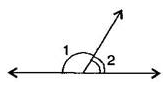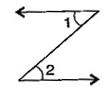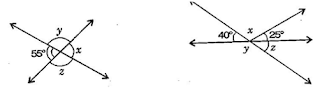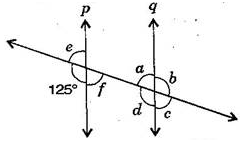# NCERT Solutions for Class 7 Maths Chapter 5 Lines and Angles| PDF Download

NCERT Solutions for Class 7 Maths Chapter 5 Lines and Angles presented here will make student confident and understanding the key concepts clearly. Here you can also download PDF of Chapter 5 Lines and Angles Class 7 Maths NCERT Solutions that will make you able to solve the difficult problems in a given in a exercise. These NCERT Solutions are prepared as per the accordance of latest CBSE guidelines so you can score maximum marks.

NCERT Solutions for Class 7 is one of the best way through which one can get command over the concepts given in the chapter. It is also useful for competitive exams and higher grades.Page No: 101

Exercise 5.1

1. Find the complement of each of the following angles:Complementary angle = 90° - given angle
(i) Complement of 20° = 90°-20° = 70°
(ii) Complement of 63° =  90° - 63° = 27°
(iii) Complement of 57° = 90°- 57° = 33°

2. Find the supplement of each of the following angles:Supplementary angle = 180° -  given angle
(i) Supplement of 105° = 180° -105° = 75°
(ii) Supplement of 87° = 180° - 87° = 93°
(iii) Supplement of 154° = 180° - 154° = 26°

3. Identify which of the following pairs of angles are complementary and which are supplementary: (i) 65°, 115°
(ii) 63°, 27°
(iii) 112°, 68°
(iv) 130∘, 50°
(v) 45°, 45°
(vi) 80°, 10°

If sum of two angles is 180°, then they are called supplementary angles. If sum of two angles is 90°, then they are called complementary angles.
(i) 65°+115° =180° These are supplementary angles.
(ii) 63°+27° =90° These are complementary angles.
(iii) 112° + 68° =180° These are supplementary angles.
(iv) 130°+50° =180° These are supplementary angles.
(v) 45° +45° =90° These are complementary angles.
(vi) 80°+10° =90°
These are complementary angles.

4. Find the angle which is equal to its complement:

Let one of the two equal complementary angles be x.
∴ x + x  = 90°
⇒ 2x = 90°
⇒x=  90°/2 = 45°
Thus, 45° is equal to its complement.

5. Find the angle which is equal to its supplement.

Let x be two equal angles of its supplement.
Therefore, x + x = 180° [Supplementary angles]
⇒ 2x = 180 ⇒ x = 180°/2 = 90°
Thus, 90° is equal to its supplement.

6. In the given figure, ∠1 and ∠2 are supplementary angles. If ∠1 is decreased, what changes should take place in ∠2 so that both the angles still remain supplementary?If ∠1 is decreased then, ∠2 will increase with the same measure, so that both the angles still remain supplementary.

7. Can two angles be supplementary if both of them are:
(i) acute
(ii) obtuse
(iii) right?

(i) No, because sum of two acute angles is less than 180°

(ii) No, because sum of two obtuse angles is more than 180°

(iii) Yes, because sum of two right angles is 180°

8. An angle is greater than 45°. Is its complementary angle greater than 45°  or equal to 45°  or less than 45°?

Let the complementary angles be x and y i.e., x + y = 90°
It is given that  x >45°
Adding y both sides, x+ y > 45° + y
⇒ 90° >  45°+ y
⇒ 90° - 45° > y
⇒ y < 45°
Thus, its complementary angle is less than 45°

Is ∠1 adjacent to ∠2? Is ∠AOC adjacent to ∠AOE? Do ∠COE and ∠EOD form a linear pair? Are ∠BOD and ∠DOA supplementary? Is ∠1 vertically opposite to ∠4? What is the vertically opposite angle of ∠5?

(i) Yes, in ∠AOE, OC is common arm.
(ii) No, they have no non-common arms on opposite side of common arm.
(iii) Yes, they form linear pair.
(iv) Yes, they are supplementary.
(v) Yes, they are vertically opposite angles.
(vi) Vertically opposite angles of ∠5 is ∠COB.

10. Indicate which pairs of angles are:
Vertically opposite angles? Linear pairs?

(i) Vertically opposite angles, ∠1, ∠4; ∠5, ∠2 + ∠3.
(ii) Linear pairs ∠1, ∠5; ∠5, ∠4.

11. In the following figure, is ∠1 adjacent to ∠2? Give reasons.∠1 and ∠2 are not adjacent angles because their vertex is not common.

12. Find the values of the angles x,y and z in each of the following:(i) x = 55°  [Vertically opposite angles]
Now 55° +  y = 180°[Linear pair]
⇒ y = 180° - 55° = 125°
Also, y=z=125° [Vertically opposite angles]
Thus, x=55°,y=125° and z=125°.

(ii) 40°+x+25°=180° [Angles on straight line]
⇒ 65° +x=180°
⇒ x=180°−65°= 115°
Now, 40°+y=180° [Linear pair]
⇒ y=180°−40°=140° ....(i)
Also, y+z=180° [Linear pair]
⇒ 140°+z=180° [From eq. (i)]
⇒ z=180°−140°=40°
Thus, x=115°,y=140° and z=40°

13. Fill in the blanks:
1. If two angles are complementary, then the sum of their measures is _______.
2. If two angles are supplementary, then the sum of their measures is __________.
3. Two angles forming a linear pair are ___________.
4. If two adjacent angles are supplementary, they form a _________.
5. If two lines intersect a point, then the vertically opposite angles are always _____.
6. If two lines intersect at a point and if one pair of vertically opposite angles are acute angles, then the other pair of vertically opposite angles are ____________.

(i) 90°
(ii) 180°
(iii) supplementary
(iv) linear pair
(v) equal
(vi) obtuse angles

14. In the adjoining figure, name the following pairs of angles:1. Obtuse vertically opposite angles.
3. Equal supplementary angles.
4. Unequal supplementary angles.
5. Adjacent angles that d° n°t f°rm a linear pair.

(i) Obtuse vertically opposite angles means greater than 90° and equal ∠AOD = ∠BOC.

(ii) Adjacent c°mplementary angles means angles have common vertex, common arm, non-common arms are on either side of common arm and sum of angles is 90°.

(iii) Equal supplementary angles means sum of angles is 180° and supplement angles are equal.

(iv) Unequal supplementary angles means sum of angles is 180° and supplement angles are unequal. i.e., ∠AOE, ∠EOC; ∠AOD, ∠DOC and ∠AOB, ∠BOC

(v) Adjacent angles that do not form a linear pair mean, angles have common ray but the angles in a linear pair are not supplementary. i.e., ∠AOB, ∠AOE; ∠AOE, ∠EOD and ∠EOD, ∠COD

Page No. 108

1. State the property that is used in each of the following statements:1. If a∥b, then ∠1 = ∠5.
2. If ∠4 = ∠6, then a∥b.

3. If ∠4 + ∠5 + 180∘, then a∥b.

(i) Given, a∥ba∥b then ∠1 = ∠5 [Corresponding angles]
If two parallel lines are cut by a transversal, each pair of corresponding angles are equal in measure.

(ii) Given, ∠4 = ∠6, then a∥b [Alternate interior angles]
When a transversal cuts two lines such that pairs of alternate interior angles are equal, the lines have to be parallel.

(iii) Given, ∠4 + ∠5 = 180∘, then a∥b [Co-interior]
When a transversal cuts two lines, such that pairs of interior angles on the same side of transversal are supplementary, the lines have to be parallel.

2. In the adjoining figure, identify:
1. the pairs of corresponding angles.
2. the pairs of alternate interior angles.
3. the pairs of interior angles on the same side of the transversal.
4. the vertically opposite angles.

(i) The pairs of corresponding angles:
∠1, ∠5; ∠2, ∠6; ∠4, ∠8 and ∠3, ∠7
(ii) The pairs of alternate interior angles are:
∠3, ∠5 and ∠2, ∠8
(iii) The pair of interior angles on the same side of the transversal:
∠3, ∠8 and ∠2, ∠5
(iv) The vertically opposite angles are:
∠1, ∠3; ∠2, ∠4; ∠6, ∠8 and ∠5, ∠7

3. In the adjoining figure, p∥q. Find the unknown angles.Given, p∥q and cut by a transversal line.
∵ 125 ̊+e=180 ̊ [Linear pair]
∴ e=180 ̊−125 ̊=55 ̊ ….(i)
Now e=f=55 ̊ [Vertically opposite angles]
Also a=f=55 ̊ [Alternate interior angles]
a+b=180 ̊ [Linear pair]
⇒ 55 ̊+b=180 ̊ [From eq. (i)]
⇒ b=180 ̊−55 ̊=125 ̊
Now a=c=55 ̊ and b=d=125 ̊ [Vertically opposite angles]
Thus, a=55 ̊,b=125 ̊,c=55 ̊,d=125 ̊,e=55 ̊ and f=55 ̊.

4. Find the values of x in each of the following figures if l∥m.(i) Given, l∥m and t is transversal line.
∴ Interior vertically opposite angle between lines ll and t=110 ̊.
∴ 110 ̊+x=180 ̊ [Supplementary angles]
⇒ x=180 ̊−110 ̊=70 ̊

(ii) Given, l∥m and t is transversal line.
x+2x=180 ̊ [Interior opposite angles]
⇒ 3x=180 ̊
⇒ x=180 ̊/3=60 ̊

(iii) Given, l∥m and a∥b
x=100 ̊ [Corresponding angles]

5. In the given figure, the arms of two angles are parallel. If ΔABC = 70 ̊, then find:(i) DGC
(ii) DEF

(i) Given, AB ∥ DE and BC is a transversal line and ∠ABC=70 ̊
∵ ∠ABC = ∠DGC [Corresponding angles]
∴ ∠DGC = 70 ̊ ….(i)

(ii) Given, BC ∥ EF and DE is a transversal line and ∠DGC=70 ̊
∵ ∠DGC = ∠DEF [Corresponding angles]
∴ ∠DEF = 70 ̊ [From eq. (i)]

6. In the given figures below, decide whether ll is parallel to m.(i) 126 ̊+44 ̊=170 ̊
ll is not parallel to mm because sum of interior opposite angles should be 180∘.

(ii) 75 ̊+75 ̊=150 ̊
ll is not parallel to mm because sum of angles does not obey the property of parallel lines.

(iii) 57 ̊+123 ̊=180 ̊
ll is parallel to mm due to supplementary angles property of parallel lines.

(iv) 98 ̊+72 ̊=170 ̊
ll is not parallel to mm because sum of angles does not obey the property of parallel lines.

## NCERT Solutions for Class 7 Maths Chapter 5 Lines and Angles

These NCERT Solutions are helpful resources that can help you not only cover the entire syllabus but also inculcate correct learning habits among students. A line has no end points on either side. A line-segment has two end points. An angle is formed when two lines (or rays or line-segments) meet.

• When lines drawn on a sheet of paper do not meet, however far produced, we call them to be parallel lines.

• When two lines intersect we have two pairs of opposite angles. They are called vertically opposite angles. They are equal in measure.

If you want to find specific question then you can find them in exercisewise NCERT Solutions which are given below. These Class 7 Maths NCERT Solutions will help you in solving the difficulties that lie ahead with ease.

NCERT Solutions given here are prepared by Studyrankers experts through which you can clear your doubts and get basic understanding of the chapter. It will be really useful in identifying, analyzing, and then rectifying the mistakes.

### NCERT Solutions for Class 7 Maths Chapters:

 Chapter 1 Integers Chapter 2 Fractions and Decimals Chapter 3 Data Handling Chapter 4 Simple Equations Chapter 6 The Triangle and its Properties Chapter 7 Congruence of Triangles Chapter 8 Comparing Quantities Chapter 9 Rational Numbers Chapter 10 Practical Geometry Chapter 11 Perimeter and Area Chapter 12 Algebraic Expressions Chapter 13 Exponents and Powers Chapter 14 Symmetry Chapter 15 Visualising Solid Shapes

FAQ on Chapter 5 Lines and Angles

#### How many exercises are there in Chapter 5 Simple Equations Class 7 Maths NCERT Solutions?

There are only two exercises in the Chapter 5 Lines and Angles which will serve as beneficial tool that can be used to recall various questions any time. These NCERT Solutions will help you get a deeper understanding of various topics.

#### What do you mean by transversal?

A line that intersects two or more lines at distinct points is called a transversal. When two lines are intersected by a transversal, pairs of corresponding angles, alternate angles and interior angles are formed.

#### What do you mean by corresponding angles?

Angles formed on the same side of a transversal, on the same side of the two lines and at corresponding vertices are called corresponding angles.

#### What do you mean by adjacent angles?

Adjacent angles have no common interior points. The angles in a linear pair are supplementary. Two adjacent angles can be either supplementary or complementary. If two adjacent angles are supplementary, then they form a straight angle, and are also called a linear pair.